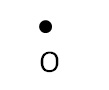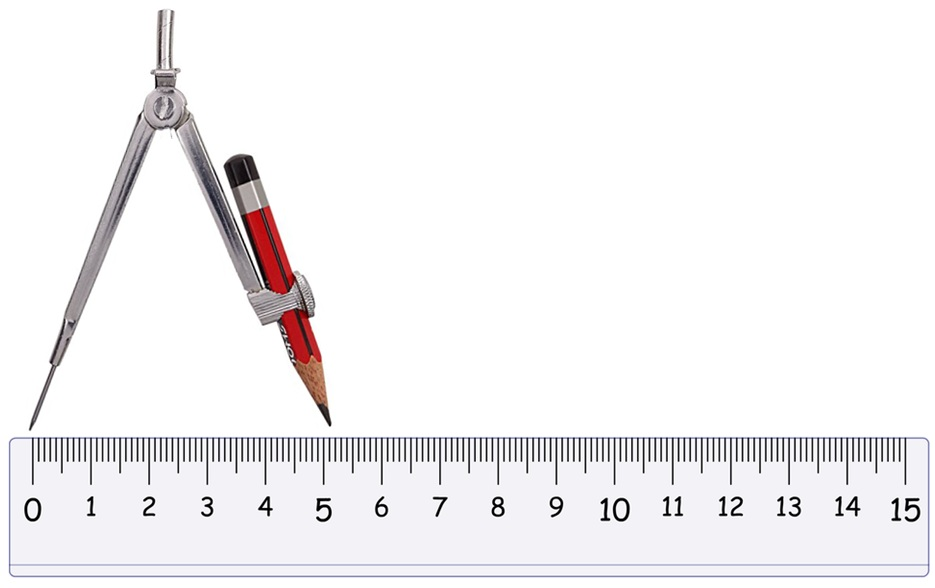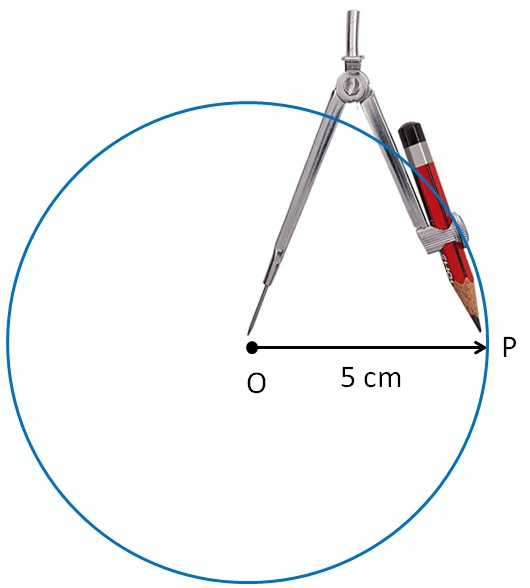Construction of a circle when its radius is known

Practical Geometry
Concept wise

Let’s take an example

#### Draw a circle of radius 5 cm

1. Mark point O as center2. Since radius is 5 cm,

we measure 5 cm using ruler and compass1. Now keeping compass opened the same length.
We keep pointed end at the center,
and draw a circle using the pencil end of the compass.So, this is the required circle with center O

and radius OP = 5 cm

Learn in your speed, with individual attention - Teachoo Maths 1-on-1 Class